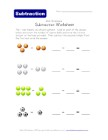Help kids learn subtraction with these picture subtraction worksheets. We have a variety of options that make it easy for kids to learn subtraction by subtracting the pictures of things like vehicles, balls, fruits, shapes, etc and writing the simple subtraction equations. These are the perfect compliment to any preschool or kindergarten math lesson plan.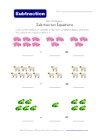## Subtraction Equations Worksheet - Animals Theme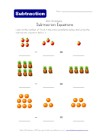## Subtraction Equations Worksheet - Fruit Theme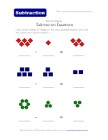## Subtraction Equations Worksheet - Shapes Theme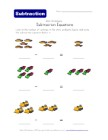## Subtraction Equations Worksheet - Vehicles Theme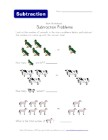## Subtraction Problems Worksheet - Animals Theme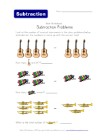## Subtraction Problems Worksheet - Musical Instruments Theme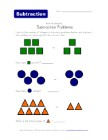## Subtraction Problems Worksheet - Shapes Theme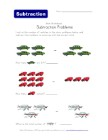## Subtraction Problems Worksheet - Vehicles Theme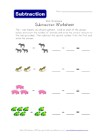## Subtraction Worksheet - Animals Theme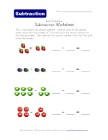## Subtraction Worksheet - Fruit Theme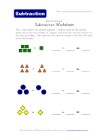## Subtraction Worksheet - Shapes Theme